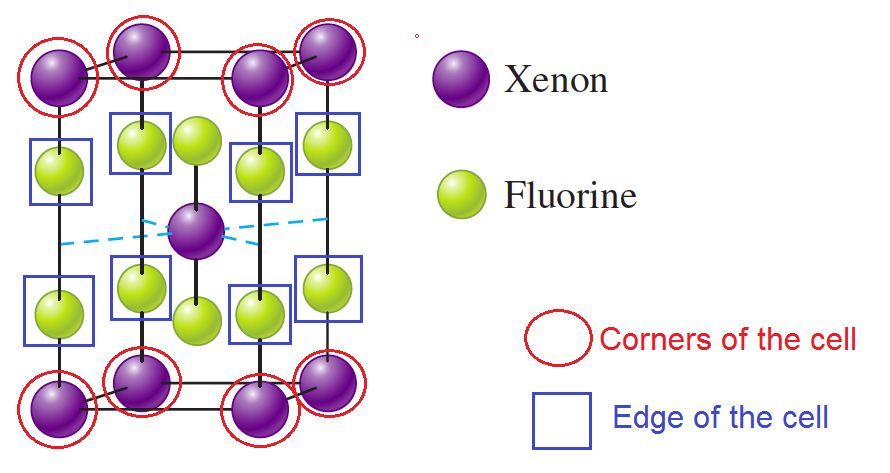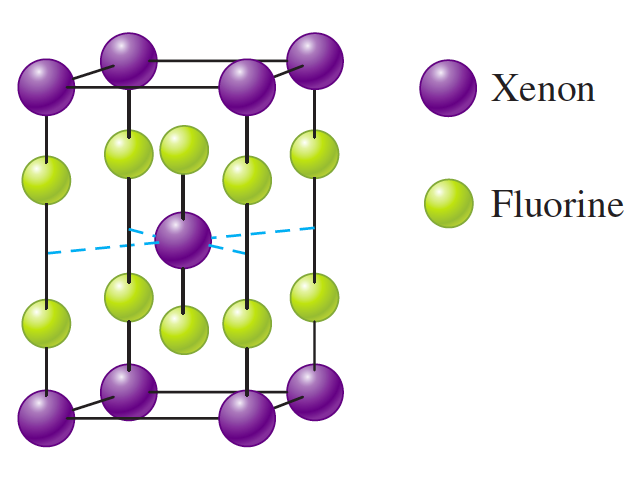# Problem: The unit cell for a pure xenon fluoride compound is shown below. What is the formula of the compound?

###### FREE Expert Solution
• Unit cell is the repeating unit of a crystal. This tells what the composition of the entire lattice system

• From given diagram, Xe atoms are located at the 8 corners and 1 in the center of the unit cell.

• On the other hand, 8 O atoms are present at the edges with 2 Os inside the cell• Each corner contributes 1/8th atom to the unit cell and each edge is 1/4 of the unit cell. Number of Xe and O atoms in 1 unit cell can be calculated as:

88% (481 ratings)###### Problem Details

The unit cell for a pure xenon fluoride compound is shown below. What is the formula of the compound?